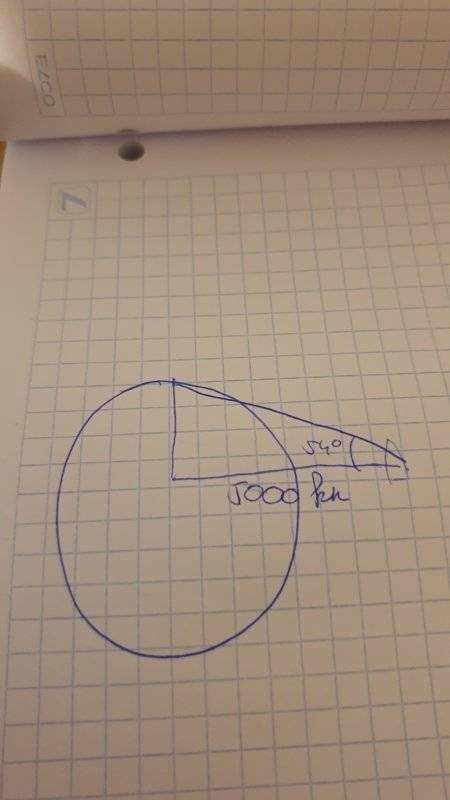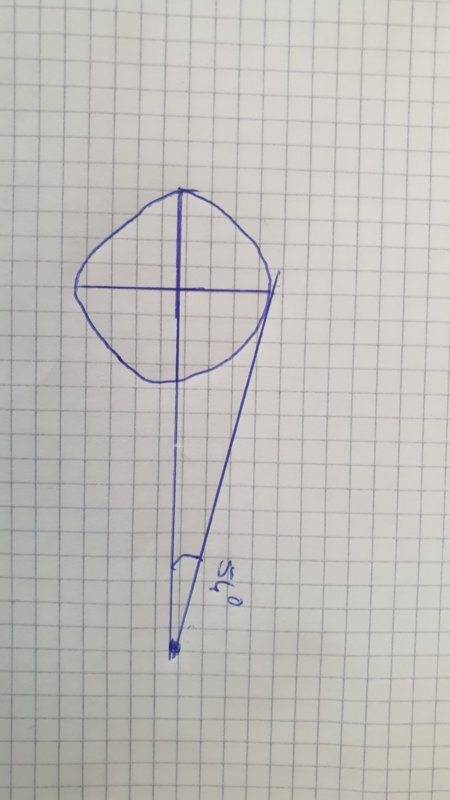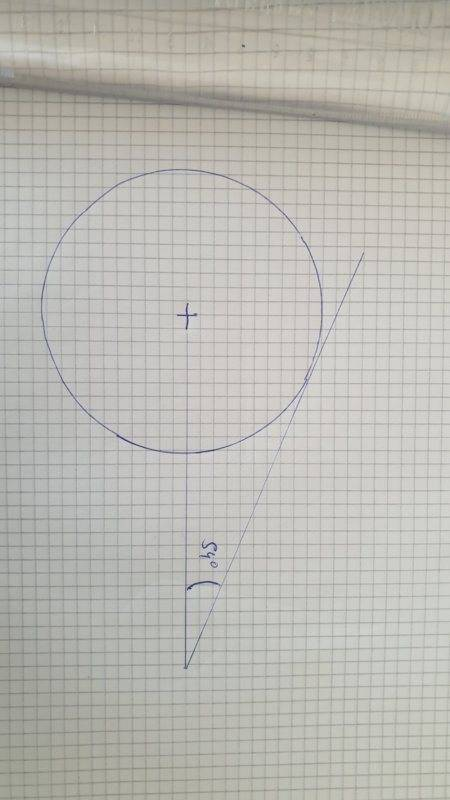# Physics problems with Newton's law of gravitation

albertus50
Homework Statement:
Hello everyone! i recently got an exercise that i have been struggling to complete.

Here it is: An astronaut weighing 74kg /163 pounds is in his space shuttle, 3106.856 miles / 5000kms from the center of an unknown planet. He proceeds to weigh himself with a scale, and he weighs 15kgs / 33 pounds. From his shuttle, he sees the planet at an angle of 54 degrees.

A) what is the radius of the planet?

b) what is the weight of the planet?

c) How much is the force of gravity at its surface?
Relevant Equations:
I think that the equation i need to use is newton's law of gravitation ( F =Gx(Mm/r^2)). and trigonometry for the 1st. However, you can use any equation as long as it solves the problem and i will do the necessary work to understand it :)
I do not know how to do this because in order to find the force of gravity it seemed to me i need to know the mass of the planet and vice versa, and for the 1st exercice i do not know whether the planet fills the astronaut's field of view. If it does in understand that i need to use trigonometry to find the radius, using 54 degrees as the angle between the x-axis (the astronaut looking perpendicularly at the center of the planet) and the top of the planet.

Staff Emeritus
Homework Helper
Gold Member
My advice: Throw away whatever textbook this question was posed in. Whatever the scale shows is going to be dependent on on the thrust of the rocket - not the gravitation of the planet.

However, part A is just geometry. Can you draw a picture that shows what the astronaut sees?

•SammyS
Homework Helper
Gold Member
2022 Award
Hmm. I'm not sure this question makes much sense to me. I assume the space shuttle is not in orbit. Is the shuttle stationary with respect to the planet? And, I'm not sure about the relevance of ##54## degrees. A planet's gravitational field should be spherically symmetric.

Is there a diagram?

Homework Helper
Gold Member
2022 Award
However, part A is just geometry. Can you draw a picture that shows what the astronaut sees?

Ah, so the planet subtends an angle of 54 degrees in his field of vision!

Staff Emeritus
Homework Helper
Gold Member
Ah, so the planet subtends an angle of 54 degrees in his field of vision!
This is the only reasonable interpretation I can imagine (well, the 54 degrees may be from horizon to horizon or centre to horizon ...)

albertus50Hello, first off thank you for taking the time to answer ! Sorry about this bad drawing, but in this case i imagine all i need to use is trigonometry in order to find the value of the side opposite of the angle. However,now that i know the radius i still do not know how to find the mass or the force of gravitation on that planet. I was thinking maybe dividing the force of gravity on Earth by ~5 since 74 divided by 5 is approximately the 15kgs that the astronaught weighs in orbit?

Homework Helper
Gold Member
2022 Award
Hello, first off thank you for taking the time to answer ! Sorry about this bad drawing, but in this case i imagine all i need to use is trigonometry in order to find the value of the side opposite of the angle. However,now that i know the radius i still do not know how to find the mass or the force of gravitation on that planet. I was thinking maybe dividing the force of gravity on Earth by ~5 since 74 divided by 5 is approximately the 15kgs that the astronaught weighs in orbit?

I guess you have translated the problem. Really we should be talking about the mass of the astronaut and the mass of the planet. You could say, I guess, that the scales are calibrated for Earth's surface gravity and record a force equivalent to that of a ##15kg## mass on the Earth's surface.

The astronaut is not in a free-falling orbit (he/she would be weightless). I think you have to assume that the shuttle is stationary relative to the planet.

There should be no problem using the gravitational force law with the data you have. Can you show an attempt to do this?

Homework Helper
Gold Member
2022 Award
PS Hint: you will need to look up either ##G##, the universal gravitational constant, or the mass and radius of the Earth.

Homework Helper
View attachment 260311Hello, first off thank you for taking the time to answer ! Sorry about this bad drawing, but in this case i imagine all i need to use is trigonometry in order to find the value of the side opposite of the angle. However,now that i know the radius i still do not know how to find the mass or the force of gravitation on that planet. I was thinking maybe dividing the force of gravity on Earth by ~5 since 74 divided by 5 is approximately the 15kgs that the astronaught weighs in orbit?

This picture is incorrect, as you can see if you study it closely. You've drawn a ray which is the hypotenuse of a right triangle. But notice that in order to reach the far vertex of that particular triangle, your ray has to penetrate the surface of the planet.

That is not the ray that defines the horizon.

The ray with the widest angle makes a tangent with the circle. And do you recall a theorem about tangents to circles and the radius from the center to where the tangent touches?

Also my interpretation is that the 54 degrees is from top to bottom, so the angle you've drawn would be only half of that, or 27 degrees.

•PeroK
Homework Helper
Gold Member
2022 Award
View attachment 260311Hello, first off thank you for taking the time to answer ! Sorry about this bad drawing, but in this case i imagine all i need to use is trigonometry in order to find the value of the side opposite of the angle. However,now that i know the radius i still do not know how to find the mass or the force of gravitation on that planet. I was thinking maybe dividing the force of gravity on Earth by ~5 since 74 divided by 5 is approximately the 15kgs that the astronaught weighs in orbit

I think your diagram is still wrong, as explained in post #9.

To find the mass of the planet let's see some calculations.

albertus50
Hello, thank you all again for answering! First off, for my equation with Newton's law of gravity i now know the answer since the only information missing out now is my mass of the planet that i want to find! My problem was i did not know the F in the
F=G x (Mp x ma)/r^2, now to isolate my M all i need to do is F x r^2 /G x ma = Mp. Thank you!

For the diagram, i would be able to find the radius with a line tangent to the planet but only if i knew the coordinates of the center of the planet, except i do not know it, maybe there is a theorem or formula i am missing out?

Homework Helper
Gold Member
2022 Award
Hello, thank you all again for answering! First off, for my equation with Newton's law of gravity i now know the answer since the only information missing out now is my mass of the planet that i want to find! My problem was i did not know the F in the
F=G x (Mp x ma)/r^2, now to isolate my M all i need to do is F x r^2 /G x ma = Mp. Thank you!

For the diagram, i would be able to find the radius with a line tangent to the planet but only if i knew the coordinates of the center of the planet, except i do not know it, maybe there is a theorem or formula i am missing out?

Try drawing the diagram carefully. Draw a proper circle (with the centre at the centre!) and draw a line from the shuttle to the surface. Do not let your line go through the surface of the planet. It doesn't need to be to scale, but it does need to be done carefully.

albertus50i would do it like this, this time my hypothenuse does not cross inside the planet. Should i still solve this using trigonometry?

•PeroK
Homework Helper
Gold Member
2022 Award
You're wasting our time with a diagram like that!

albertus50is this any better?

Homework Helper
Gold Member
2022 Award
That's better. As mentioned above, I suspect the half angle you have shown should be ##27## degrees.

(The one you have drawn looks close to ##27## degrees.)

You need to add the radius of the planet and use the key fact about a radius and a tangent to the same point on the circle.

•